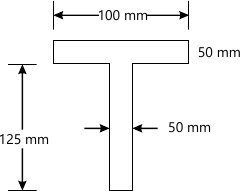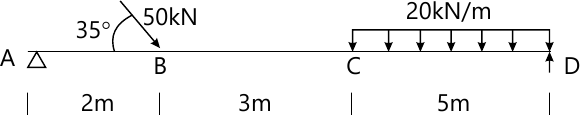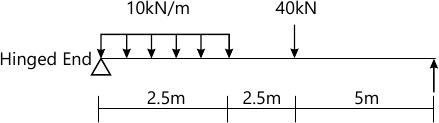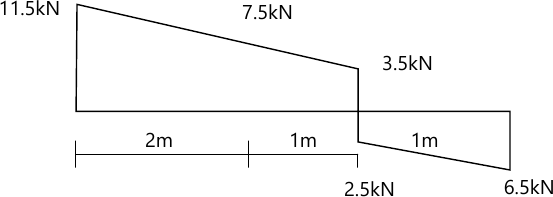MORE IN Strength of Materials
SPPU Civil Engineering (Semester 3)
Strength of Materials
May 2015
Total marks: --
Total time: --
INSTRUCTIONS
(1) Assume appropriate data and state your reasons
(2) Marks are given to the right of every question
(3) Draw neat diagrams wherever necessary

Answer any one question from Q1 and Q2
1 (a) A Compound bar ABC 1.5 m long is made up of two parts 'AB' of aluminium and 'BC' of steel having cross-sectional area of steel half of the aluminium bar. The rod is fixed at 'A' and subjected to an axial pull of 200 kN at end 'C'. If the elongations of both materials is equal, find the lengths of each part assuming Esteel=200 Gpa and Ealuminium as one third of steel.
6 M
1 (b) A simply supported beam 8m span carrier u.d.l of 3 kN/m over entire span. Find the maximum bending stress induced if the cross-section is as shown in Fig. 16 M

2 (a) A steel bar 2 m long is at 30°C. The temperature of the rod is increased by 150°C. Find :
i) Free expansion of the rod
ii) temperature stress produced if expansion is prevented and nature of the stress.
iii) stress produced if 2.5 mm expansion is permitted. Assume supports are unyielding? Take E=210 Gpa, and α=12×10-6/°C. Assume bar diameter = 16 mm.
6 M
2 (b) An I section has the following dimensions. Web: 300 mm×10mm, Flange 150mm × 20 mm. The maximum shear stress developed in the beam is 14.8 Mpa. Find the shear force to which the beam is subjected.
6 M

3 (a) Find maximum torque that can be safely applied to a shaft of 80 mm diameter. The permissible angle of twist is 1.5 degree in a length of 5 m and shear stress not to exceed 42 Mpa. Take G=84 Mpa.
6 M
Answer any one question from Q3 and Q4
3 (b) A shaft of 95 mm diameter transmits 200 kW power at 100 rpm. If at a section bending moment is 15 kNm, then find the principal stress, maximum shear stress.
6 M

4 (a) A steel rod 28mm in diameter is 3.5 m long. Find the maximum instantaneous stress induced and work done at maximum elongation when a load of 80 kN is suddenly applied. Take E=210 Gpa.
6 M
4 (b) A hollow shaft having an inside diameter 60% of its outer is to replace a solid shaft transmitting the same power at same speed. Calculate the percentage saving in material if material to be used is also the same.
6 M

Answer any one question from Q5 and Q6
5 (a) An overhanging beam ABC with end 'A' hinged and simply supported at 'B' is loaded with udl of intensity 30 kN/m acting on 2 m length from 'A?and a point load of 10 kN acting at free end 'C'. Draw B.M.D. and S.F.D. Assume 1(AB)=4 m and 1(BC)=1m.
7 M
5 (b) Draw shear force diagram, bending moment diagram and axial thrust diagram for the beam ABCD with end 'A' hinged and loaded as shown in Fig. 26 M

6 (a) Draw shear force diagram and bending moment diagram for the beam as shown in fig 3. Indicate the numerical values at all important section. Find the position and value of maximum bending moment.7 M
6 (b) The diagram shown in fig. 4 is the shear force diagram for a beam which rests on two supports, one being at the left hand end. No couple is acting on beam.6 M

Answer any one question from Q7and Q8
7 (a) Compare the crippling loads given by the Euler's and Rankine's formula for a circular column of 40 mm diameter and 2000m long. Take yield as 300 Mpa. Rankine's constant a=1/7500 and E=200 Gpa.
6 M
7 (b) State assumptions made in Euler's theory and its limitations.
7 M

8 (a) Explain core of the section and hence obtain a core section for a hollow circular column of external and internal diameter 'D?and 'd' respectively.
6 M
8 (b) A hollow rectangular section is having external size 500 mm × 450 mm and internal size 400 mm × 350 mm. It carries a vertical load of 100 kN at the outer edge of the column on X-axis. Calculate maximum and minimum intensities of stress in the section. Assume 500 mm side horizontal.
7 M

More question papers from Strength of Materials